# Class 11 Maths NCERT Solutions for Chapter 10 Straight Lines Exercise 10.3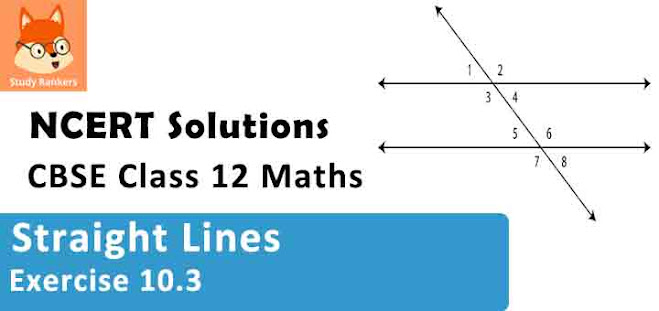### Straight Lines Exercise 10.3 Solutions

1. Reduce the following equation into slope-intercept form and find their slopes and the y-intercepts.
(i) x + 7y = 0
(ii) 6x + 3y -5 = 0
(iii) y = 0

Solution

(i) The given equation is x + 7y = 0
It can be written as
y = -(1/7)x + 0  ...(1)
This equation is of the form y = mx + c, where m = -1/7 and c = 0.
Therefore, equation (1) is in the slope-intercept form, where the slope and the y-intercept are -1/7 and 0 respectively.

(ii) The given equation is 6x + 3y - 5 = 0
It can be written as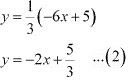This equation is of the form y = mx + c, where m = -2 and c = 5/3.
Therefore, equation (2) is in the slope-intercept form, where the slope and the y-intercept are–2 and 5/3 respectively.

(iii) The given equation is y = 0.
It can be written as
y = 0.x + 0 …(3)
This equation is of the form y = mx + c, where m = 0 and c = 0.
Therefore, equation (3) is in the slope-intercept form, where the slope and the y-intercept are 0 and 0 respectively.

2. Reduce the following equations into intercept form and find their intercepts on the axes.
(i) 3+ 2– 12 = 0
(ii) 4– 3= 6
(iii) 3+ 2 = 0.

Solution

(i) The given equation is  3x + 2y - 12 = 0.
It can be written as:
3x + 2y = 12
3x/12 + 2y/12 =1
i.e., x/4 + y/6 = 1 ...(1)
This equation is of the form x/a + y/b = 1, where a = 4 and b = 6.
Therefore, equation (1) is in the intercept form, where the intercepts on the and axes are 4 and 6 respectively.

(ii) The given equation is 4– 3= 6.
It can be written as:
4x/6 - 3y/6 = 1
2x/3 - y/2 = 1
i.e., x/(3/2) + y/(-2) = 1 ...(2)
This equation is of the form x/a + y/b = 1, where a = 3/2 and b = -2.
Therefore, equation (2) is in the intercept form, where the intercepts on the x and y axes are 3/2 and –2 respectively.

(iii) The given equation is 3+ 2 = 0.
It can be written as:
3y = -2
i.e., y/(-2/3) = 1 ...(3)
This equation is of the form x/a + y/b = 1 , where a = 0 and b = -2/3 .
Therefore, equation (3) is in the intercept form, where the intercept on the y - axis is  -2/3 and it has no intercept on the x - axis.

3. Reduce the following equations into normal form. Find their perpendicular distances from the origin and angle between perpendicular and the positive x-axis.
(i) x - √3y + 8 = 0
(ii) – 2 = 0
(iii) – = 4

Solution

(i) The given equation is x - √3y + 8 = 0
It can be reduced as:
x - √3y = -8
⇒ -x + √3y = 8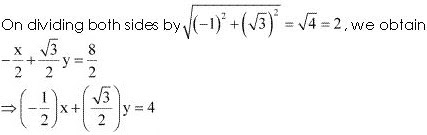⇒ x cos  120° + y sin 120° = 4 ...(1)
Equation (1) is in the normal form.
On comparing equation (1) with the normal form of equation of line
x cos ω + y sin ω = p, we obtain ω = 120° and p = 4.
Thus, the perpendicular distance of the line from the origin is 4, while the angle between the perpendicular and the positive x-axis is 120°.

(ii) The given equation is y – 2 = 0.
It can be reduced as 0.x + 1.y = 2
On dividing both sides by √(02 + 12 ) = 1, we obtain 0.x + 1.y = 2
⇒ x cos 90° + y sin 90° = 2 …(1)
Equation (1) is in the normal form.
On comparing equation (1) with the normal form of equation of line
x cos ω + y sin ω = p, we obtain ω = 90° and p = 2.
Thus, the perpendicular distance of the line from the origin is 2, while the angle between the
perpendicular and the positive x-axis is 90°.

(iii) The given equation is x – y = 4.
It can be reduced as 1.x + (–1) y = 4
On dividing both sides by √1[2 + (-1)2] = √2, we obtain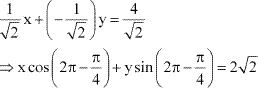⇒ x cos 315° + y sin 315° = 2√2  ...(1)
Equation (1) is in the normal form.
On comparing equation (1) with the normal form of equation of line
x cos ω + y sin ω = p, we obtain ω = 315° and p = 2√2 .
Thus, the perpendicular distance of the line from the origin is 2√2, while the angle between the perpendicular and the positive x-axis is 315°.

4. Find the distance of the point (–1, 1) from the line 12(+ 6) = 5(– 2).
Solution
The given equation of the line is 12(x + 6) = 5(y – 2).
⇒ 12x + 72 = 5y – 10
⇒12x – 5y + 82 = 0 … (1)
On comparing equation (1) with general equation of line Ax + By + C = 0, we obtain A = 12, B = –5, and C = 82.
It is known that the perpendicular distance (d) of a line Ax + By + C = 0 from a point (x1, y1) is given by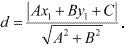The given point is (x1y1) = (–1, 1).
Therefore, the distance of point (–1, 1) from the given line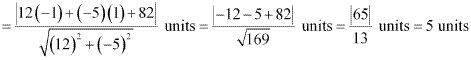5. Find the points on the x - axis, whose distances from  the x/3 + y/4 = 1 are 4 units.

Solution

The given equation of line is
x/3 + y/4 = 1
or, 4x + 3y - 12 = 0  ...(1)
On comparing equation (1) with general equation of line Ax By C = 0, we obtain A = 4, B = 3, and C = –12.
Let (a, 0) be the point on the x-axis whose distance from the given line is 4 units.
It is known that the perpendicular distance (d) of a line Ax By C = 0 from a point (x1y1) is given byTherefore,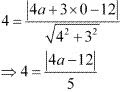⇒ |4a - 12|  = 20
⇒ ± (4a - 12) = 20
⇒ (4a - 12) = 20 or -(4a - 12) = 20
⇒ 4a = 20 + 12 or 4a = -20 + 12
⇒ a = 8 or -2
Thus, the required points on the x - axis are (-2, 0) and (8, 0).

6. Find the distance between parallel lines
(i) 15x + 8y – 34 = 0 and 15x + 8y + 31 = 0
(ii) l (x + y) + p = 0 and l (x + y) – r = 0

Solution

It is known that the distance (d) between parallel lines Ax + By + C1 = 0 and Ax + By + C2 = 0 is given by(i) The given parallel lines are 15x + 8y – 34 = 0 and 15x + 8y + 31 = 0.
Here, A = 15, B = 8, C1 = –34, and C= 31.
Therefore, the distance between the parallel lines is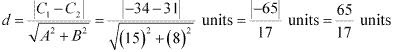(ii) The given parallel lines are l (x + y) + p = 0 and l (x + y) – r = 0.
lx + ly + p = 0 and lx + ly – r = 0
Here, A = l, B = l, C1 = p, and C= – r.
Therefore, the distance between the parallel lines is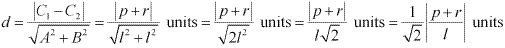7. Find equation of the line parallel to the line 3– 4y + 2 = 0 and passing through the point (–2, 3).

Solution

The equation of the given line is
3x - 4y + 2 = 0
or y = 3x/4 + 2/4
or y = 3x/4 + 1/2 , which is of the form y = mx + c
∴ Slope of the given line = 3/4
It is known that parallel lines have the same slope.
∴ Slope of the other line = m = 3/4
Now, the equation of the line that has a slope of 3/4 and passes through the point (-2, 3) is
(y - 3) = 3/4 {x - (-2)}
4y - 12 = 3x + 6
i.e., 3x - 4y + 18 = 0

8. Find equation of the line perpendicular to the line – 7+ 5 = 0 and having intercept 3.

Solution

The given equation of line is  x - 7y + 5 = 0
Or, y = (1/7)x  + 5/7 , which is of the form y = mx + c
∴ Slope of the given line = 1/7
The slope of the line perpendicular to the line having a slope of 1/7 is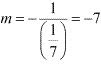The equation of the line with slope –7 and x-intercept 3 is given by
y = m (x – d)
⇒ y = –7 (x – 3)
⇒ y = –7x + 21
⇒ 7x + y = 21

9. Find angles between the lines √3x + y = 1 and x + √3y = 1 .

Solution

The given lines are √3x + y = 1 and x + √3y = 1
y = -√3x + 1    ....(1) and y = -(1/√3)x + 1/√3  ...(2)
The slope of line (1), is m1 = -√3 , while the slope of line (2) is m2 = -(1/√3) .
The acute angle i.e., θ between the two lines is given by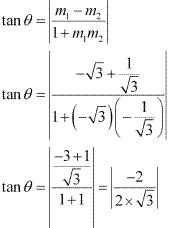tan θ = 1/√3
θ = 30°
Thus, the angle between the given lines is either 30° or 180°- 30° = 150°.

10. The line through the points (h, 3) and (4, 1) intersects the line 7x – 9y – 19 = 0. at right angle. Find the value of h.

Solution

The slope of the line passing through points (h, 3) and (4, 1) is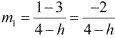The slope of line 7x - 9y - 19 = 0 y = (7/9)x - (19/9) is m2 = 7/9.
It is given that the two lines are perpendicular.
∴ m1 × m2 = -1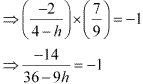⇒ 14 = 36 - 9h
⇒ 9h = 36 - 14
⇒ h = 22/9
Thus, the value of h is 22/9.

11. Prove that the line through the point (x1y1) and parallel to the line Ax + By + C = 0 is A (x –x1B (y – y1) = 0.

Solution

The slope of line Ax + By + C = 0 or y = -(A/B)x + -(C/B) is m = -(A/B)
It is known that parallel lines have the same slope.
∴ Slope of the other line  = m = -(A/B)
The equation of the line passing through point (x1, y1 )and having a slope m = -(A/B) is
y - y1 = m(x - x1)
⇒ y - y1  = -(A/B) (x - x1
⇒ B(y -y1) = -A(x - x1
⇒ A(x - x1) + B(y - y1) = 0
Hence, the line through point (x1, y1) and parallel to line Ax + By + C = 0 is A(x - x1)+ B(y - y1) = 0.

12. Two lines passing through the point (2, 3) intersects each other at an angle of 60°. If slope of one line is 2, find equation of the other line.

Solution

It is given that the slope of the first line, m1 = 2.
Let the slope of the other line be m2.
The angle between the two lines is 60°.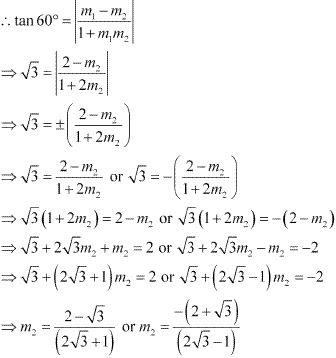Case I: m2 = (2 - √3)/(2√3 + 1)
The equation of the line passing through point (2, 3) and having a slope of
(2 - √3)/(2√3 + 1) is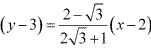⇒ (2√3 + 1)y - 3(2√3 + 1) = (2 - √3)x - 2(2 - √3)
⇒ (√3 - 2)x + (2√3 + 1)y = -4 + 2√3 + 6√3 + 3
⇒ (√3 - 2)x + (2√3 + 1)y = -1 + 8√3
In this case, the equation of the other line is (√3 - 2)x + (2√3 + 1)y = -1 + 8√3.

Case II :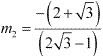The equation of the line passing through point (2, 3) and having a slope  of  -(2 + √3)/(2√3 - 1) is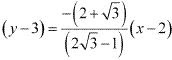⇒ (2√3 - 1)y - 3(2√3 - 1) = -(2 + √3)x + 2(2 + √3)
⇒ (2√3 - 1)y + (2 + √3)x = 4 + 2√3 + 6√3 - 3
⇒ (2 + √3)x + (2√3 - 1)y = 1 + 8√3
In this case, the equation of the other line is (2 + √3)x + (2√3 - 1)y = 1 + 8√3.
Thus, the required equation of the other line is (√3 - 2)x + (2√3 + 1)y = -1 + 8√3 or (2 + √3)x + (2√ - 1)y = 1 + 8√3.

13. Find the equation of the right bisector of the line segment joining the points (3, 4) and (1, 2).

Solution

The right bisector of a line segment bisects the line segment at 90°.
The end-points of the line segment are given as A (3, 4) and B (–1, 2).
Accordingly, mid-point of AB = [(3-1)/2, (4+2)/2] = (1, 3)
Slope of AB  = (2 -4)/(-1-3) = -2/-4 = 1/2
∴ Slope of the line perpendicular to AB = -1/(1/2) = -2
The equation of the line passing through (1, 3) and having a slope of –2 is
(y – 3) = –2 (x – 1)
⇒ y – 3 = –2x + 2
⇒ 2x + y = 5
Thus, the required equation of the line is 2x + y = 5.

14. Find the coordinates of the foot of perpendicular from the point (1, 3) to the line 3– 4– 16 = 0.

Solution

Let (ab) be the coordinates of the foot of the perpendicular from the point (–1, 3) to the line 3x – 4y – 16 = 0.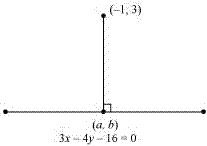Slope of the line joining (-1, 3) and (a, b), m1 = (b - 3)/(a + 1)
Slope of the line 3x - 4y - 16 = 0 or y = (3/4)x - 4, m2 = 3/4
Since these two lines are perpendicular, m1 m2 = -1⇒ 3b - 9 = -4a - 4
⇒ 4a + 3b = 5  ...(1)
Point (a, b) lies on line 3x - 4y = 16.
∴ 3a - 4b = 16  ...(2)
On solving equations (1) and (2), we obtain
a = 68/25 and b = 49/25
Thus, the required coordinates of the foot of the perpendicular are (68/25, -49/25).

15. The perpendicular from the origin to the line y = mx + c meets it at the point (1, 2). Find the values of and c.

Solution

The given equation of line is y = mx + c.
It is given that the perpendicular from the origin meets the given line at (–1, 2).
Therefore, the line joining the points (0, 0) and (–1, 2) is perpendicular to the given line.
∴Slope of the line joining (0, 0) and (–1, 2) = 2/-1 = -2
The slope of the given line is m
∴ m × -2 = -1 [The two lines are perpendicular]
⇒ m = 1/2
Since point (-1, 2) lies on the given line, it satisfies the equation y = mx + c.
∴ 2 = m(-1) + c
⇒ 2 = (1/2) (-1) + c
⇒ c = 2 + 1/2 = 5/2
Thus, the respective values of m and c are 1/2 and 5/2.

16. If p and q are the lengths of perpendiculars from the origin to the lines x cos θ – y sin θ = k cos 2θ and xsec θ+ y cosec θ = k, respectively, prove that p2 + 4q2 = k2

Solution

The equations of given lines are
x cos θ – y sinθ = k cos 2θ …(1)
x secθ + y cosec θ= k …(2)
The perpendicular distance (d) of a line Ax + By + C = 0 from a point (x1, y1) is given byOn comparing equation (1) to the general equation of line i.e., Ax By C = 0, we obtain A = cosθB = –sinθ, and C = –k cos 2θ.
It is given that is the length of the perpendicular from (0, 0) to line (1).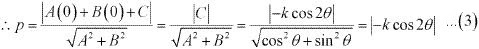On comparing equation (2) to the general equation of line i.e., Ax + By + C = 0, we obtain A = secθ, B = cosecθ, and C = ­–k.
It is given that q is the length of the perpendicular from (0, 0) to line (2).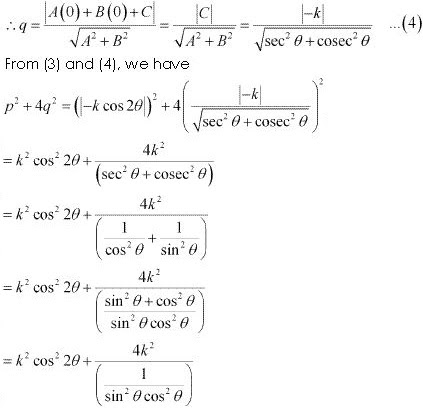= k2 cos2 2θ + 4k2 sin2 θ cos2 θ
= k2 cos2 2θ + k2 (2 sin θ cos θ)2
= k2 cos2 2θ + k2 sin2 2θ
= k2 ( cos2 2θ + sin2 2θ)
= k2
Hence, we proved that p2 + 4q2 = k2

17. In the triangle ABC with vertices A (2, 3), B (4, 1) and C (1, 2), find the equation and length of altitude from the vertex A.

Solution

Let AD be the altitude of triangle ABC from vertex A.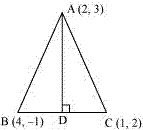The equation of the line passing through point (2, 3) and having a slope of 1 is
(y – 3) = 1(x – 2)
⇒ x – y + 1 = 0
⇒ – x = 1
Therefore, equation of the altitude from vertex A = – x = 1.
Length of AD = Length of the perpendicular from A (2, 3) to BC
The equation of BC is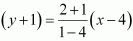⇒ (y + 1) = -1(x - 4)
⇒ y + 1 = -x + 4
⇒ x + y - 3 = 0  ...(1)
The perpendicular distance (d) of a line Ax + By + C = 0 from a point (x1, y1) is given byOn comparing equation (1) to the general equation of line Ax + By + C = 0 , we obtain A = 1, B = 1, and C = -3.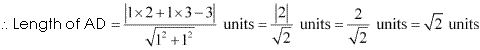Thus, the equation and the length of the altitude from vertex A are y - x = 1 and √2 units respectively.

18. If p is the length of perpendicular from the origin to the line whose intercepts on the axes are a and b, then show that 1/p2 = 1/a2 + 1/b2

Solution

It is known that the equation of a line whose intercepts on the axes are a and b is
x/a + y/b = 1
or bx + ay = ab
or bx + ay - ab = 0  ...(1)
The perpendicular distance (d) of a line Ax + By + C = 0 from a point  (x1, y1) is given byOn comparing equation (1) to the general equation of line Ax + By + C = 0, we obtain A = b, B = a, and C = –ab.
Therefore, if p is the length of the perpendicular from point (x1, y1) = (0, 0) to line (1), we obtain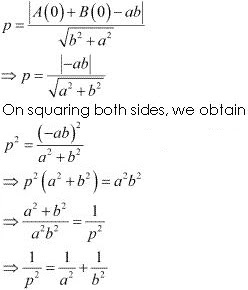Hence, we showed that 1/p2 = 1/a2 + 1/b2.